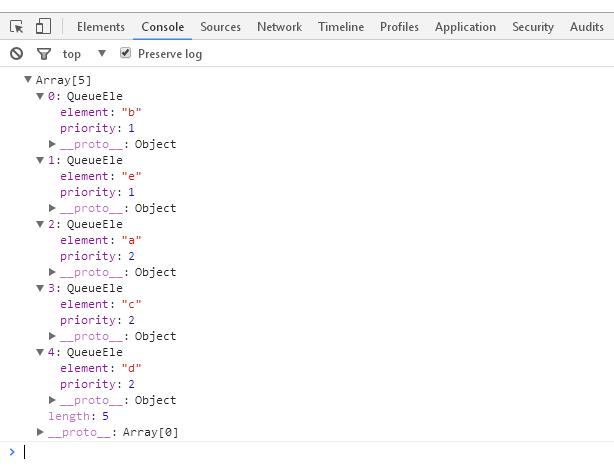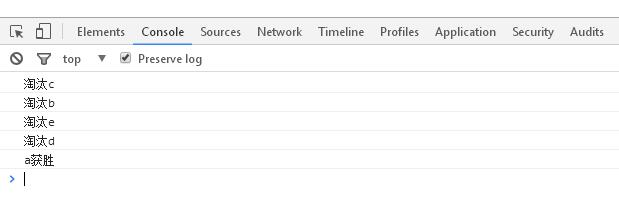# JavaScript數據結構之優先隊列與循環隊列實例詳解

```//創建一個類來表示優先隊列
function Priorityqueue(){
var items=[];//保存隊列里的元素
function QueueEle(e,p){//元素節點，有兩個屬性
this.element=e;//值
this.priority=p;//優先級
}
this.enqueue=function(e,p){//添加一個元素到隊列尾部
var queueEle=new QueueEle(e,p);
//priority小的優先級高，優先級高的在隊頭
if(this.isEmpty()){
items.push(queueEle);
}else{
for(var i=0;i<items.length;i++){
if(items[i].priority>queueEle.priority){
items.splice(i,0,queueEle);
break;
}
}
items.push(queueEle);
}
}
}
this.isEmpty=function(){
return items.length==0;
}
this.dequeue=function(){
return items.shift();
}
this.clear=function(){
items=[];
}
this.print=function(){
console.log(items);
}
this.mylength=function(){
return items.length;
}
}
var pqueue=new Priorityqueue();
pqueue.enqueue('a',2);
pqueue.enqueue('b',1);
pqueue.enqueue('c',2);
pqueue.enqueue('d',2);
pqueue.enqueue('e',1);
pqueue.print();
//[ QueueEle { element: 'b', priority: 1 },
// QueueEle { element: 'e', priority: 1 },
// QueueEle { element: 'a', priority: 2 },
// QueueEle { element: 'c', priority: 2 },
// QueueEle { element: 'd', priority: 2 } ]

``````//創建一個類來表示隊列
function Queue(){
var items=[];//保存隊列里的元素
this.enqueue=function(e){//添加一個元素到隊列尾部
items.push(e);
}
this.dequeue=function(){//移除隊列的第一項，并返回
return items.shift();
}
this.front=function(){//返回隊列的第一項
return items;
}
this.isEmpty=function(){//如果隊列中部包含任何元素，返回true，否則返回false
return items.length==0;
}
this.mylength=function(){//返回隊列包含的元素個數
return items.length;
}
this.clear=function(){//清除隊列中的元素
items=[];
}
this.print=function(){//打印隊列中的元素
console.log(items);
}
}
//擊鼓傳花
function hotPotato(namelist,num){
var queue=new Queue();
for(var i=0;i<namelist.length;i++){
queue.enqueue(namelist[i]);
}
var eliminated='';
while(queue.mylength()>1){
for(i=0;i<num;i++){
queue.enqueue(queue.dequeue());
}
eliminated=queue.dequeue();
console.log("淘汰"+eliminated);
}
return queue.dequeue();
}
var namelist=['a','b','c','d','e'];
var winner=hotPotato(namelist,7);
console.log(winner+"獲勝");
//淘汰c
//淘汰b
//淘汰e
//淘汰d
//a獲勝

```JS獲取數組中出現次數最多及第二多元素的方法

Summernote是一個基于jquery的bootstrap超級簡單WYSIWYG在線編輯器。Summernote非常的輕量級，大小只有30KB，支持Safari,Chrome,Firefox、Opera、InternetExplorer9+(IE8支持即

mint-ui的search組件在鍵盤顯示搜索按鈕的實現方法